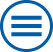## The group contributes to the following mathematical research topics of WIAS:

Interacting stochastic particle systems

In the mathematical modeling of many processes and phenomena in the Sciences and Technology one employs systems with many random particles and interactions. [>> more]

Large deviations

The theory of large deviations, a branch of probability theory, provides tools for the description of the asymptotic decay rate of a small probability, as a certain parameter diverges or shrinks to zero. Examples are large times, low temperatures, large numbers of stochastic quantities, or an approximation parameter. [>> more]

Nonlinear kinetic equations

Kinetic equations describe the rate at which a system or mixture changes its chemical properties. Such equations are often non-linear, because interactions in the material are complex and the speed of change is dependent on the system size as well as the strength of the external influences. [>> more]

Random geometric systems

Systems with many random components distributed in space (points, edges, graphs, trajectories, etc.) with many short- or long-range interactions are examined at the WIAS for their macroscopic properties. Particular attention is paid to the formation of particularly large structures in the system or other phase transitions. [>> more]

Spectral theory of random operators

Many questions about processes and phenomena are described by random operators, most notably by the random Schrödinger operator (Anderson operator) and the Laplace operator with random weights (conductances) on the edges between nearest neighbours. [>> more]

Variational methods

Many physical phenomena can be described by suitable functionals, whose critical points play the role of equilibrium solutions. Of particular interest are local and global minimizers: a soap bubble minimizes the surface area subject to a given volume and an elastic body minimizes the stored elastic energy subject to given boundary conditions. [>> more]

## Archive

### Further mathematical research topics where the institute has expertise in:

Interacting random systems

Large random interacting particle systems are mostly defined either by coupled systems of stochastic differential equations or via Hamiltonian functions. [>> more]